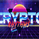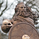8668 lượt xem
The slow relative strength index (SRSI) indicator created by Vitali Apirine is a momentum price oscillator similar to RSI in its application and interpretation. Oscillating between 0 and 100, it generates both OB/OS signals and midline (50) cross over signals and divergences.

As author suggests, bullish / bearish divergences generated by SRSI are not as effective during strong trends. To avoid fading an established trend, the system is used in conjunction with a trend confirmation tool like ADX indicator.

You can configure the OB/OS levels, default are 70/30.

The slow relative strength index , TASC 2015-07

List of my public indicators: http://bit.ly/1LQaPK8
List of my app-store indicators: http://blog.tradingview.com/?p=970

```//
// @author LazyBear
//
// List of my public indicators: http://bit.ly/1LQaPK8
// List of my app-store indicators: http://blog.tradingview.com/?p=970
//
study("Apirine Slow RSI [LazyBear]", shorttitle="ASRSI_LB", overlay=false, precision=3)
periods = input( 6, title="Smoothing", minval=1, maxval=100 )
smooth =  input( 14, title="RSI Length", minval=1, maxval=100 )
price = input(close, title="Source")
calc_wima(src, length) =>
MA_s=(src + nz(MA_s * (length-1)))/length
MA_s

r1 = ema( price, periods )
r2 = iff( price > r1, price - r1, 0 )
r3 = iff( price < r1, r1 - price, 0 )
r4 = calc_wima( r2, smooth )
r5 = calc_wima( r3, smooth )
rr = iff( r5 == 0, 100, 100 - ( 100 / ( 1 + ( r4 / r5 ) ) ) )
obl=input(70, title="OB Level")
ovl=input(30, title="OS Level")
obl1=hline(obl, title="OB", color=gray), osl1=hline(ovl, title="OS", color=gray)
fill(obl1,osl1, color=gray, transp=80, title="RegionFill")
hline(50, title="MidLine", color=gray)
plot( rr, title="SlowRSI", color=red, linewidth=2 )
```
List of my free indicators: http://bit.ly/1LQaPK8
List of my indicators at Appstore: http://blog.tradingview.com/?p=970

## Bình luậnThanks for this LB. I used your code to make a candlestick version with volume weighting and HA candle options. I hope you dont mind (i did give you credit of course).

Phản hồiLooks good LB. Thanks for sharing it and all the other indicators that you provide to us. By the way, I have looked for a Time Series Forecast indicator in the scripts and have not found it. Do you know if it exists? Thanks again.
Phản hồiFuturaman
aha, there is at least one Ehlers hater out there :)
Phản hồiLazyBear
Ehler's stuff is quite good if used with some common sense :)

BB squeeze + cgo signal after expanding range in one direction.
Phản hồi